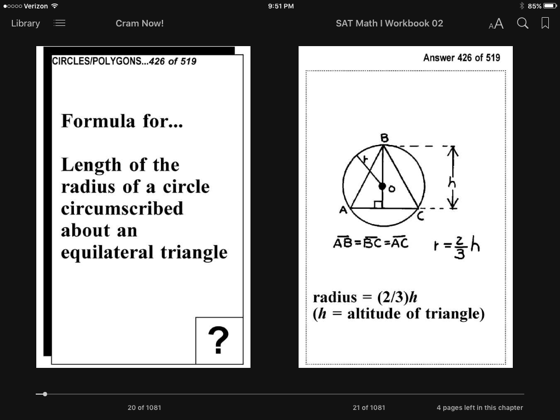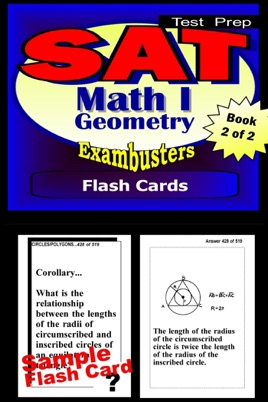• USD 4.99

## Descripción de editorial

"SAT MATH LEVEL I Prep Flashcard Workbook 2 of 2: GEOMETRY"
450 questions and answers (ILLUSTRATED). Essential definitions, formulas, and concepts with complementary diagrams. Topics: Lines and Angles, Triangles, Introduction to Proofs, Congruent Triangles, Perpendicular Lines, Parallel Lines, Angle Sums, Quadrilaterals, Medians, Altitudes, Bisectors, Circles, Ratio and Proportion, Similar Polygons, Proportions in Right Triangles, Areas of Polygons, Circles and Regular Polygons, Inequalities, Locus, Introduction to Coordinate Geometry
[==================]
"SAT MATH LEVEL I Prep Flashcard Workbook 1 of 2: ALGEBRA REVIEW"
450 questions and answers. Essential definitions, formulas, concepts, and sample problems. Topics: Sets, Variables, Exponents, Properties of Numbers, Like Terms, Simple Equations, Property of Equality, Signed Numbers, Monomials, Polynomials, Advanced Equations, Verbal Problems, Factoring Polynomials, Algebraic Fractions, Equations with Several Variables, Advanced Verbal Problems, Evaluating Formulas, Simultaneous Equations, Ratio and Proportion, Variation, Quadratic Equations and Radicals, Coordinate Geometry
=============================
"EXAMBUSTERS SAT II Prep Workbooks" provide comprehensive SAT II review--one fact at a time--to prepare students to take practice SAT II tests. Each SAT II study guide focuses on fundamental concepts and definitions--a basic overview to begin studying for the SAT II exam. Up to 600 questions and answers, each volume in the SAT II series is a quick and easy, focused read. Reviewing SAT II flash cards is the first step toward more confident SAT II preparation and ultimately, higher SAT II exam scores!

•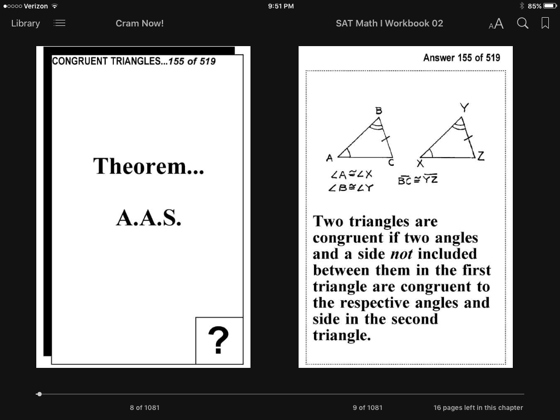•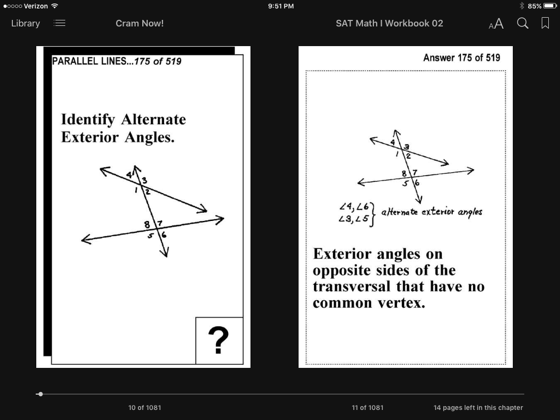•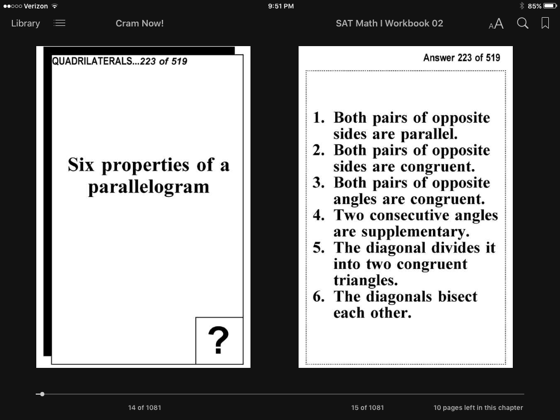•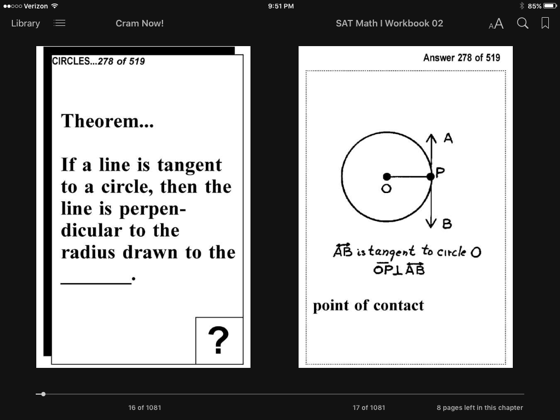•